机器学习技术在交易中的应用

Author: , Created: 2019-08-29 09:42:00, Updated: 2019-09-02 08:53:02

关于研究环境的搭建

• 安装发明者量化托管者和设置Anaconda

制定交易策略

• 方向：确定资产是否便宜,昂贵或者价值公允.

• 开仓条件：如果资产价格便宜或者昂贵，你应该做多或者做空.

• 平仓交易：如果资产价格合理且我们在该资产中持有仓位（先前的买入或卖出），你是否应平仓

• 价格范围：进行开仓交易的价格（或范围）

• 数量：交易资金的数量（例如数字货币的数量或者商品期货的手数）

策略方法

pip install -U scikit-learn

使用机器学习创建交易策略信号

• 数据挖掘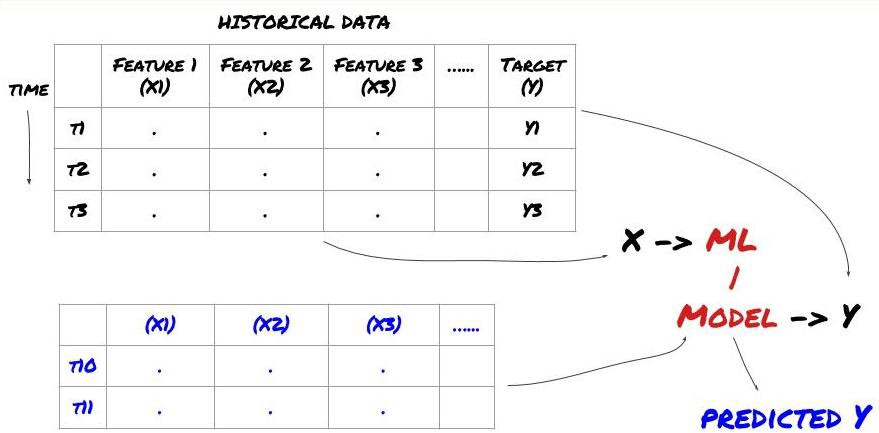第一步: 设置你的问题

• 你想要预测什么？什么是好的预测？你如何评价预测结果?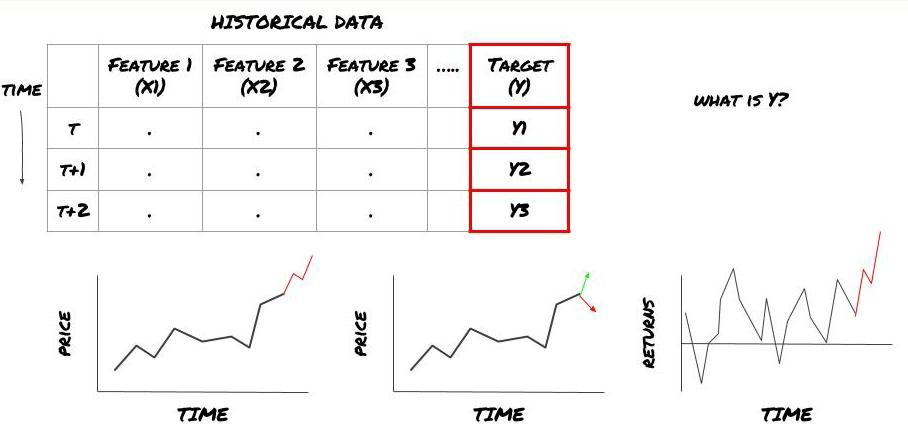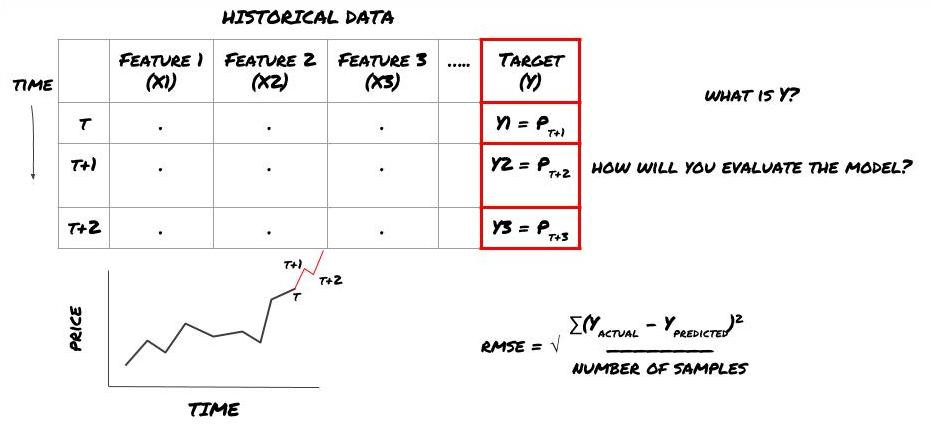basis = Price of Stock — Price of Future

basis(t)=S(t)−F(t)

Y(t) = future expected value of basis = Average(basis(t+1),basis(t+2),basis(t+3),basis(t+4),basis(t+5))

• 我们的目标：创建一个模型，使预测值尽可能接近Y.

第二步：收集可靠数据

from backtester.dataSource.quant_quest_data_source import QuantQuestDataSource
cachedFolderName = '/Users/chandinijain/Auquan/qq2solver-data/historicalData/'
dataSetId = 'trainingData1'
instrumentIds = ['MQK']
ds = QuantQuestDataSource(cachedFolderName=cachedFolderName,
dataSetId=dataSetId,
instrumentIds=instrumentIds)
data = None
for key in ds.getBookDataByFeature().keys():
if data is None:
data = pd.DataFrame(np.nan, index = ds.getBookDataByFeature()[key].index, columns=[])
data[key] = ds.getBookDataByFeature()[key]
data['Stock Price'] =  ds.getBookDataByFeature()['stockTopBidPrice'] + ds.getBookDataByFeature()['stockTopAskPrice'] / 2.0
data['Future Price'] = ds.getBookDataByFeature()['futureTopBidPrice'] + ds.getBookDataByFeature()['futureTopAskPrice'] / 2.0
data['Y(Target)'] = ds.getBookDataByFeature()['basis'].shift(-5)
del data['benchmark_score']
del data['FairValue']
return data

第三步：拆分数据

• 从数据创建训练集，交叉验证和测试这些数据集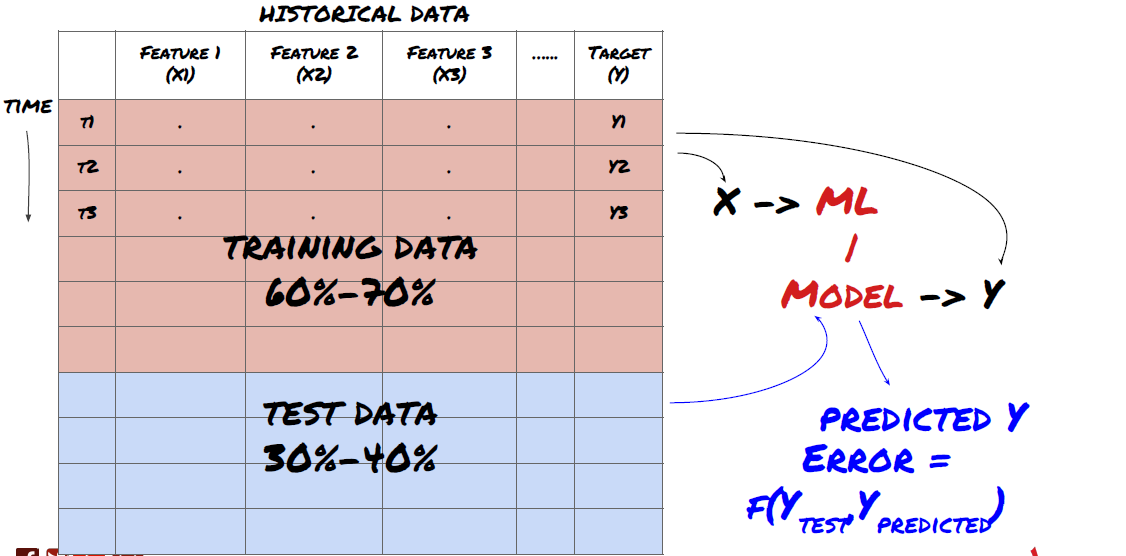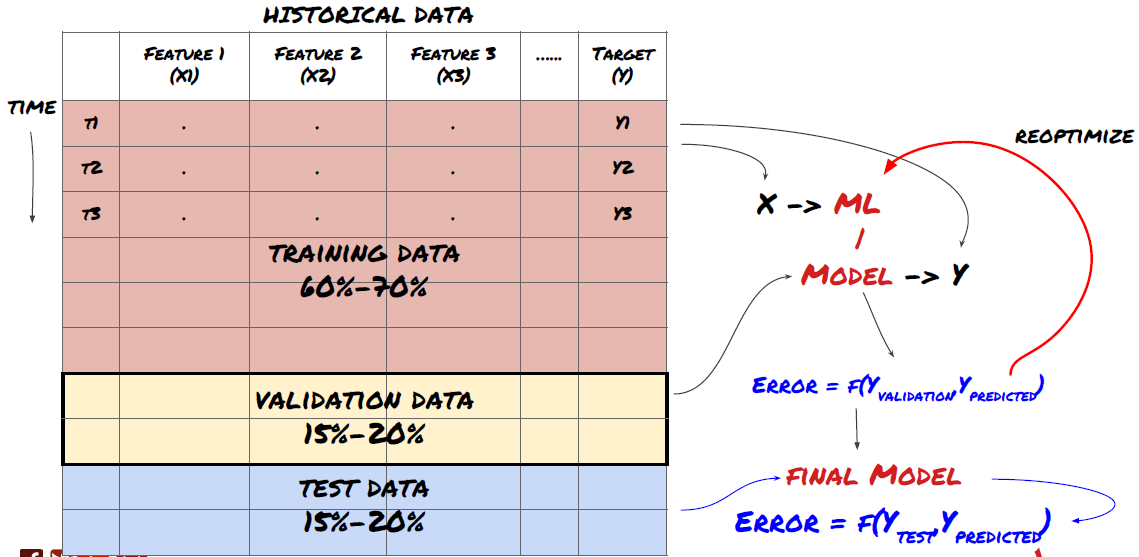# Training Data
dataSetId =  'trainingData1'
ds_training = QuantQuestDataSource(cachedFolderName=cachedFolderName,
dataSetId=dataSetId,
instrumentIds=instrumentIds)
# Validation Data
dataSetId =  'trainingData2'
ds_validation = QuantQuestDataSource(cachedFolderName=cachedFolderName,
dataSetId=dataSetId,
instrumentIds=instrumentIds)
# Test Data
dataSetId =  'trainingData3'
ds_test = QuantQuestDataSource(cachedFolderName=cachedFolderName,
dataSetId=dataSetId,
instrumentIds=instrumentIds)

def prepareData(data, period):
data['Y(Target)'] = data['basis'].rolling(period).mean().shift(-period)
if 'FairValue' in data.columns:
del data['FairValue']
data.dropna(inplace=True)
period = 5
prepareData(training_data, period)
prepareData(validation_data, period)
prepareData(out_of_sample_test_data, period)

第四步：特征工程

• 在还没有探索与目标变量的关系的情况下，不要随意选择一大组特征

• 与目标变量很少或没有关系可能会导致过度拟合

• 你选择的特征可能彼此高度相关，在这种情况下，较少数量的特征也可以解释目标

• 我通常会创建一些具有直观意义的特征，查看目标变量与这些特征的相关性，以及它们之间的相关性以决定使用哪些

• 你还可以尝试根据最大信息系数（MIC），执行主成分分析（PCA）和其他方法对候选特征进行排序

特征转换/规范化：

ML模型往往在标准化方面表现良好。但是，在处理时间序列数据时，规范化很棘手，因为未来的数据范围是未知的。你的数据可能超出标准化范围，导致模型错误。但是你仍然可以尝试强制某种程度的平稳性：

• 缩放：按标准差或四分位数范围划分特征

• 居中：从当前值中减去历史平均值

• 归一化：上述（x - mean）/ stdev的两个回溯期

• 常规归一化：在回溯期（x-min）/（max-min）内将数据标准化为-1到+1的范围并重新确定中心

def rsi(data, period):
data_upside = data.sub(data.shift(1), fill_value=0)
data_downside = data_upside.copy()
data_downside[data_upside > 0] = 0
data_upside[data_upside < 0] = 0
avg_upside = data_upside.rolling(period).mean()
avg_downside = - data_downside.rolling(period).mean()
rsi = 100 - (100 * avg_downside / (avg_downside + avg_upside))
rsi[avg_downside == 0] = 100
rsi[(avg_downside == 0) & (avg_upside == 0)] = 0
return rsi
def create_features(data):
basis_X = pd.DataFrame(index = data.index, columns =  [])

basis_X['mom3'] = difference(data['basis'],4)
basis_X['mom5'] = difference(data['basis'],6)
basis_X['mom10'] = difference(data['basis'],11)

basis_X['rsi15'] = rsi(data['basis'],15)
basis_X['rsi10'] = rsi(data['basis'],10)

basis_X['emabasis3'] = ewm(data['basis'],3)
basis_X['emabasis5'] = ewm(data['basis'],5)
basis_X['emabasis7'] = ewm(data['basis'],7)
basis_X['emabasis10'] = ewm(data['basis'],10)
basis_X['basis'] = data['basis']
basis_X['vwapbasis'] = data['stockVWAP']-data['futureVWAP']

data['stockTopBidPrice']
data['futureTopBidPrice']

basis_X['btopbid'] = data['stockTopBidPrice'] -
data['futureTopBidPrice']

basis_X['totalbidvol'] = data['stockTotalBidVol'] -
data['futureTotalBidVol']

basis_X['emabasisdi7'] = basis_X['emabasis7'] -
basis_X['emabasis5'] +
basis_X['emabasis3']

basis_X = basis_X.fillna(0)

basis_y = data['Y(Target)']
basis_y.dropna(inplace=True)

print("Any null data in y: %s, X: %s"
%(basis_y.isnull().values.any(),
basis_X.isnull().values.any()))
print("Length y: %s, X: %s"
%(len(basis_y.index), len(basis_X.index)))

return basis_X, basis_y
basis_X_train, basis_y_train = create_features(training_data)
basis_X_test, basis_y_test = create_features(validation_data)

第五步：模型选择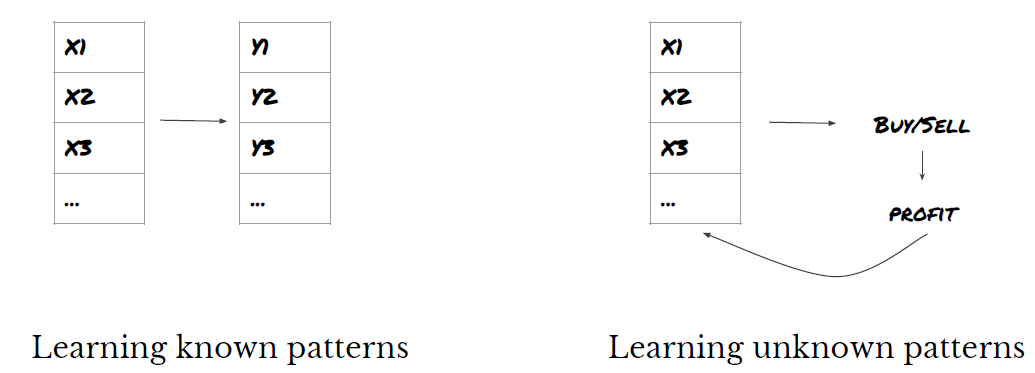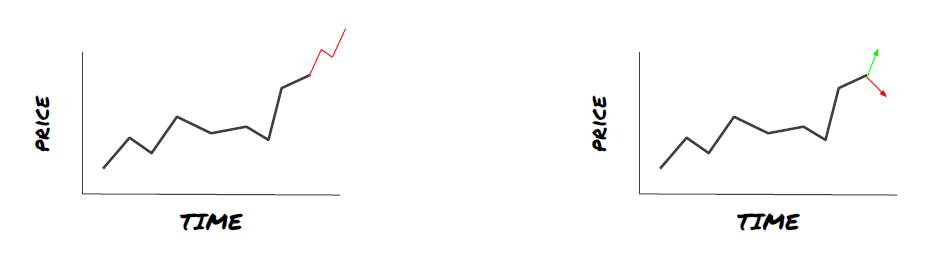• 线性回归（参数，回归）

• Logistic回归（参数，分类）

• K近邻(KNN)算法（基于实例，回归）

• SVM，SVR（参数，分类和回归）

• 决策树

• 决策森林

第六步：训练，验证和优化（重复步骤4-6）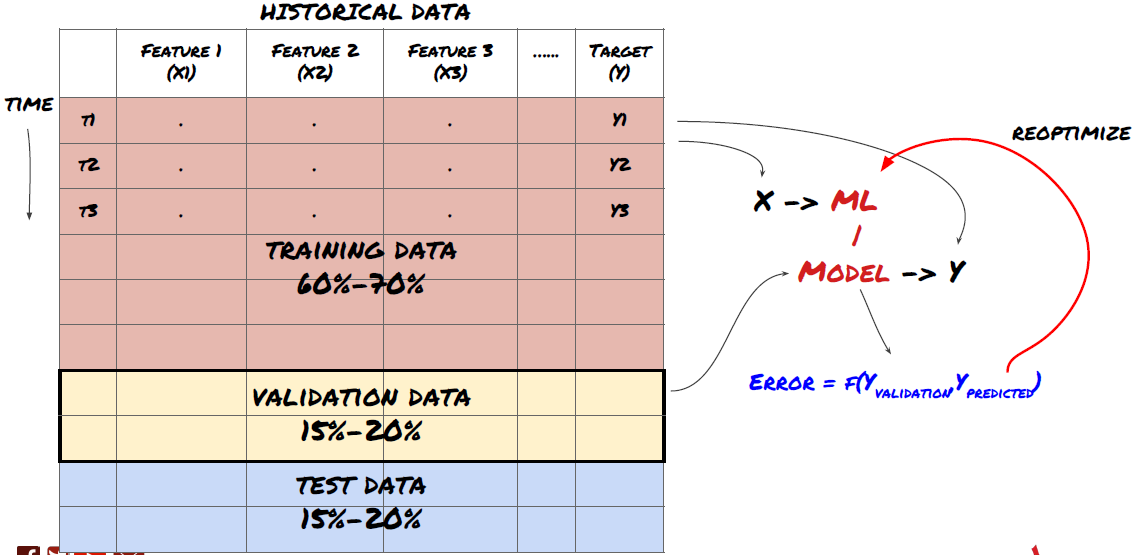from sklearn import linear_model
from sklearn.metrics import mean_squared_error, r2_score
def linear_regression(basis_X_train, basis_y_train,
basis_X_test,basis_y_test):

regr = linear_model.LinearRegression()
# Train the model using the training sets
regr.fit(basis_X_train, basis_y_train)
# Make predictions using the testing set
basis_y_pred = regr.predict(basis_X_test)
# The coefficients
print('Coefficients: \n', regr.coef_)

# The mean squared error
print("Mean squared error: %.2f"
% mean_squared_error(basis_y_test, basis_y_pred))

# Explained variance score: 1 is perfect prediction
print('Variance score: %.2f' % r2_score(basis_y_test,
basis_y_pred))
# Plot outputs
plt.scatter(basis_y_pred, basis_y_test,  color='black')
plt.plot(basis_y_test, basis_y_test, color='blue', linewidth=3)
plt.xlabel('Y(actual)')
plt.ylabel('Y(Predicted)')
plt.show()

return regr, basis_y_pred
_, basis_y_pred = linear_regression(basis_X_train, basis_y_train,
basis_X_test,basis_y_test)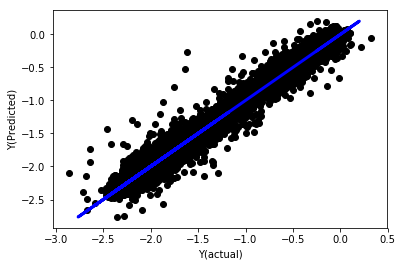('Coefficients: \n', array([ -1.0929e+08, 4.1621e+07, 1.4755e+07, 5.6988e+06, -5.656e+01, -6.18e-04, -8.2541e-05,4.3606e-02, -3.0647e-02, 1.8826e+07, 8.3561e-02, 3.723e-03, -6.2637e-03, 1.8826e+07, 1.8826e+07, 6.4277e-02, 5.7254e-02, 3.3435e-03, 1.6376e-02, -7.3588e-03, -8.1531e-04, -3.9095e-02, 3.1418e-02, 3.3321e-03, -1.3262e-06, -1.3433e+07, 3.5821e+07, 2.6764e+07, -8.0394e+06, -2.2388e+06, -1.7096e+07]))
Mean squared error: 0.02
Variance score: 0.96

def normalize(basis_X, basis_y, period):
basis_X_norm = (basis_X - basis_X.rolling(period).mean())/
basis_X.rolling(period).std()
basis_X_norm.dropna(inplace=True)
basis_y_norm = (basis_y -
basis_X['basis'].rolling(period).mean())/
basis_X['basis'].rolling(period).std()
basis_y_norm = basis_y_norm[basis_X_norm.index]

return basis_X_norm, basis_y_norm
norm_period = 375
basis_X_norm_test, basis_y_norm_test = normalize(basis_X_test,basis_y_test, norm_period)
basis_X_norm_train, basis_y_norm_train = normalize(basis_X_train, basis_y_train, norm_period)
regr_norm, basis_y_pred = linear_regression(basis_X_norm_train, basis_y_norm_train, basis_X_norm_test, basis_y_norm_test)
basis_y_pred = basis_y_pred * basis_X_test['basis'].rolling(period).std()[basis_y_norm_test.index] + basis_X_test['basis'].rolling(period).mean()[basis_y_norm_test.index]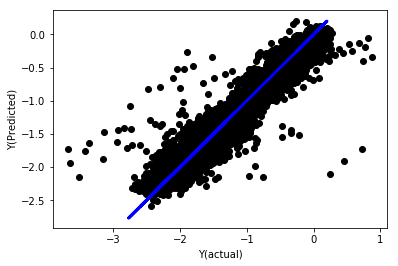Mean squared error: 0.05
Variance score: 0.90

for i in range(len(basis_X_train.columns)):
print('%.4f, %s'%(regr_norm.coef_[i], basis_X_train.columns[i]))

19.8727, emabasis4
-9.2015, emabasis5
8.8981, emabasis7
-5.5692, emabasis10
-0.0036, rsi15
-0.0146, rsi10
0.0196, mom10
-0.0035, mom5
-7.9138, basis
0.0062, swidth
0.0117, fwidth
2.0311, btopbid
0.0611, bavgbid
0.0113, topbidvolratio
0.0231, totalbidvolratio

import seaborn

c = basis_X_train.corr()
plt.figure(figsize=(10,10))
seaborn.heatmap(c, cmap='RdYlGn_r', mask = (np.abs(c) <= 0.8))
plt.show()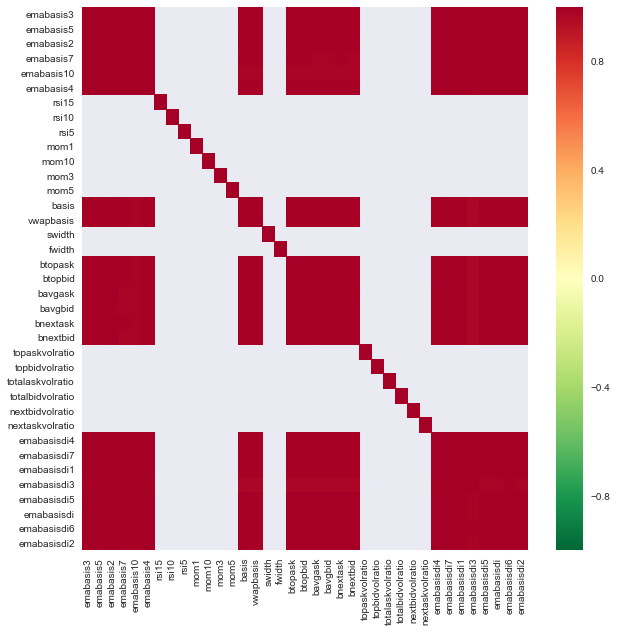def create_features_again(data):
basis_X = pd.DataFrame(index = data.index, columns =  [])
basis_X['mom10'] = difference(data['basis'],11)
basis_X['emabasis2'] = ewm(data['basis'],2)
basis_X['emabasis5'] = ewm(data['basis'],5)
basis_X['emabasis10'] = ewm(data['basis'],10)
basis_X['basis'] = data['basis']
100000
basis_X['totalbidvolratio'] = (data['stockTotalBidVol']
- data['futureTotalBidVol'])/
100000
basis_X = basis_X.fillna(0)

basis_y = data['Y(Target)']
basis_y.dropna(inplace=True)
return basis_X, basis_y
basis_X_test, basis_y_test = create_features_again(validation_data)
basis_X_train, basis_y_train = create_features_again(training_data)
_, basis_y_pred = linear_regression(basis_X_train, basis_y_train, basis_X_test,basis_y_test)
basis_y_regr = basis_y_pred.copy()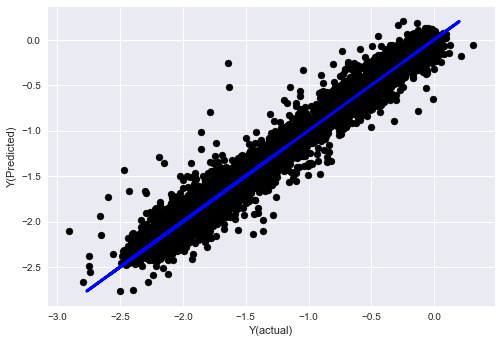('Coefficients: ', array([ 0.03246139,
0.49780982, -0.22367172,  0.20275786,  0.50758852,
-0.21510795, 0.17153884]))
Mean squared error: 0.02
Variance score: 0.96

• K近邻(KNN)算法
from sklearn import neighbors
n_neighbors = 5
model = neighbors.KNeighborsRegressor(n_neighbors, weights='distance')
model.fit(basis_X_train, basis_y_train)
basis_y_pred = model.predict(basis_X_test)
basis_y_knn = basis_y_pred.copy()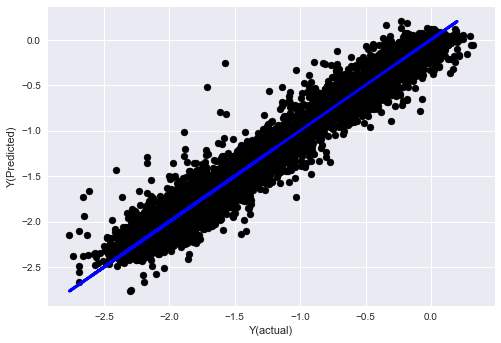• SVR
from sklearn.svm import SVR
model = SVR(kernel='rbf', C=1e3, gamma=0.1)
model.fit(basis_X_train, basis_y_train)
basis_y_pred = model.predict(basis_X_test)
basis_y_svr = basis_y_pred.copy()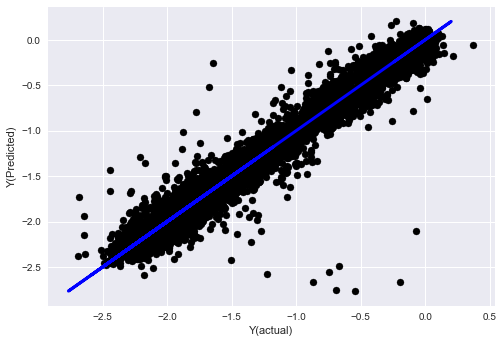• 决策树
model=ensemble.ExtraTreesRegressor()
model.fit(basis_X_train, basis_y_train)
basis_y_pred = model.predict(basis_X_test)
basis_y_trees = basis_y_pred.copy()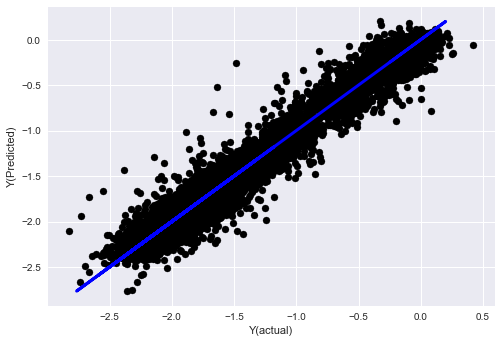第七步：对测试数据进行回测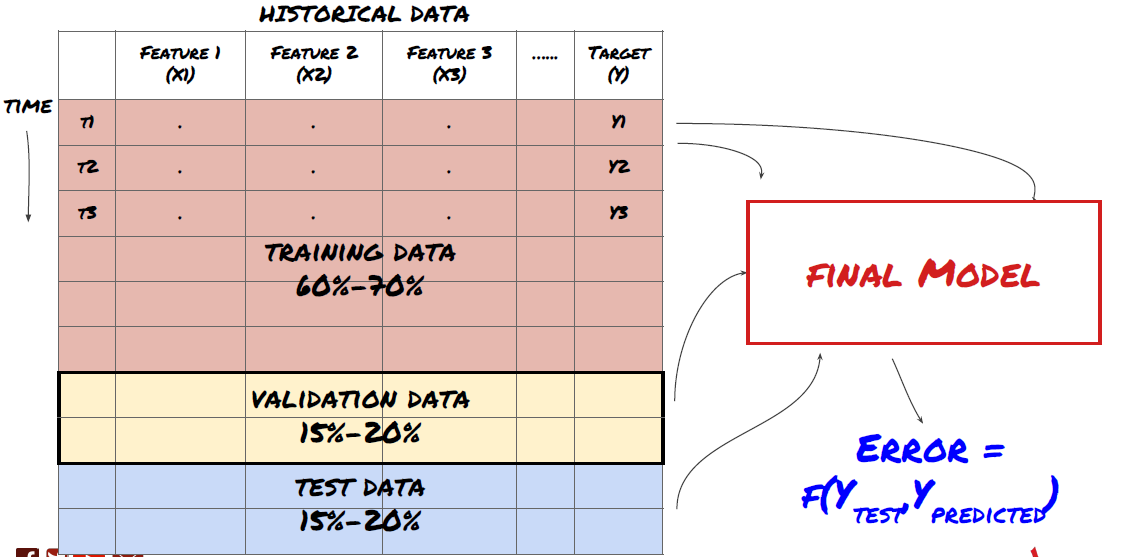import backtester
from backtester.features.feature import Feature
class Problem1Solver():
def getTrainingDataSet(self):
return "trainingData1"
return ['MQK']
def getCustomFeatures(self):
return {'my_custom_feature': MyCustomFeature}
def getFeatureConfigDicts(self):

expma5dic = {'featureKey': 'emabasis5',
'featureId': 'exponential_moving_average',
'params': {'period': 5,
'featureName': 'basis'}}
expma10dic = {'featureKey': 'emabasis10',
'featureId': 'exponential_moving_average',
'params': {'period': 10,
'featureName': 'basis'}}
expma2dic = {'featureKey': 'emabasis3',
'featureId': 'exponential_moving_average',
'params': {'period': 3,
'featureName': 'basis'}}
mom10dic = {'featureKey': 'mom10',
'featureId': 'difference',
'params': {'period': 11,
'featureName': 'basis'}}

return [expma5dic,expma2dic,expma10dic,mom10dic]

def getFairValue(self, updateNum, time, instrumentManager):
# holder for all the instrument features
lbInstF = instrumentManager.getlookbackInstrumentFeatures()
mom10 = lbInstF.getFeatureDf('mom10').iloc[-1]
emabasis2 = lbInstF.getFeatureDf('emabasis2').iloc[-1]
emabasis5 = lbInstF.getFeatureDf('emabasis5').iloc[-1]
emabasis10 = lbInstF.getFeatureDf('emabasis10').iloc[-1]
basis = lbInstF.getFeatureDf('basis').iloc[-1]
totalbidvol = lbInstF.getFeatureDf('stockTotalBidVol').iloc[-1] - lbInstF.getFeatureDf('futureTotalBidVol').iloc[-1]

coeff = [ 0.03249183, 0.49675487, -0.22289464, 0.2025182, 0.5080227, -0.21557005, 0.17128488]
newdf['MQK'] = coeff * mom10['MQK'] + coeff * emabasis2['MQK'] +\
coeff * emabasis5['MQK'] + coeff * emabasis10['MQK'] +\
coeff * basis['MQK'] + coeff * totalaskvol['MQK']+\
coeff * totalbidvol['MQK']

newdf.fillna(emabasis5,inplace=True)
return newdf
problem1Solver = Problem1Solver()
shouldPlot=True,
makeInstrumentCsvs=False)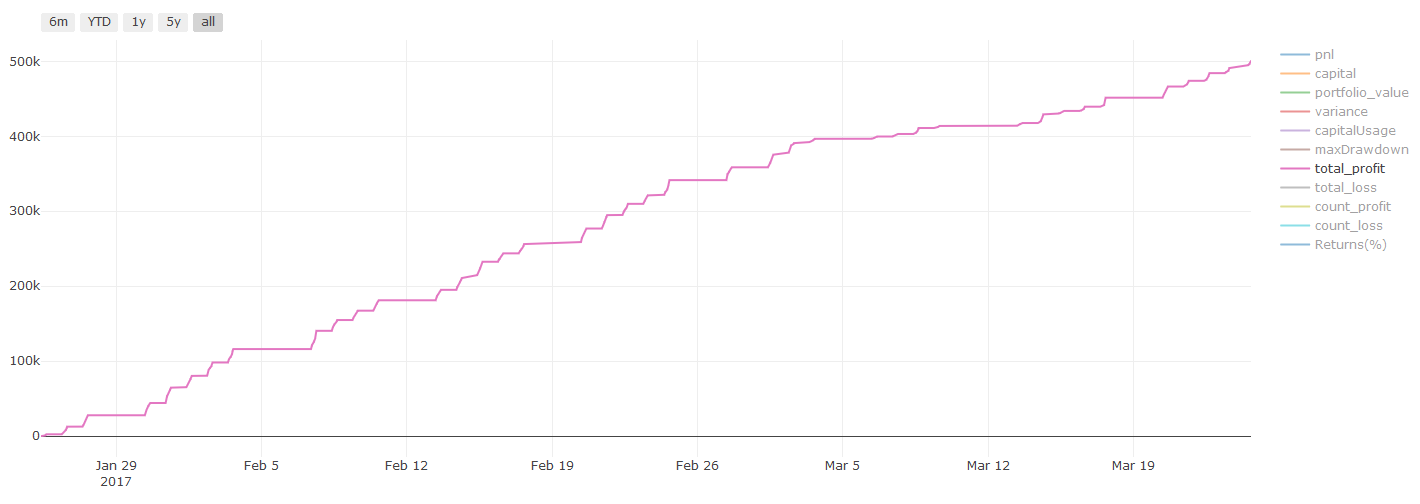第八步：改进模型的其他方法

1. 滚动验证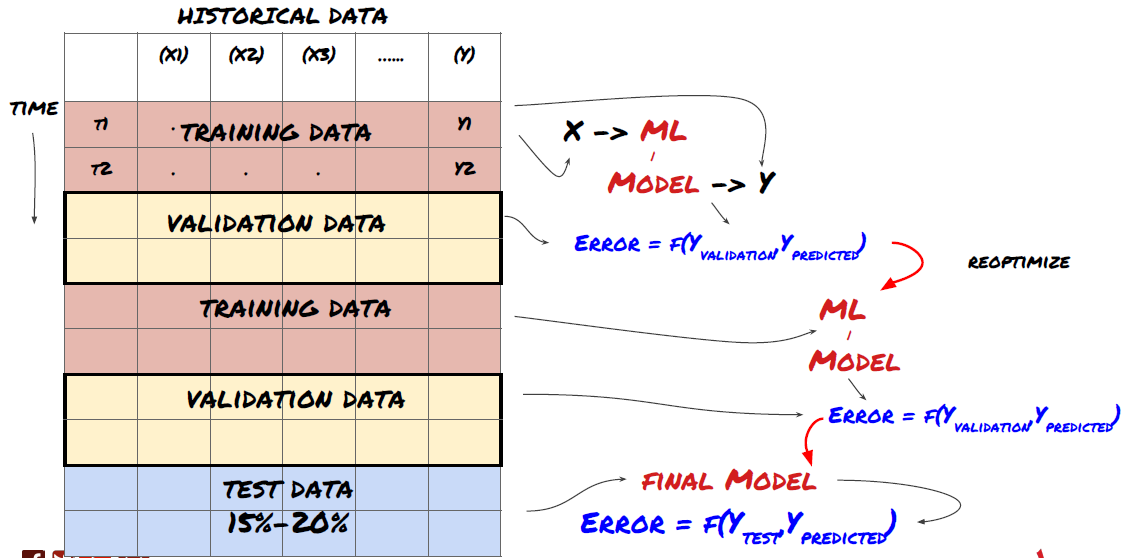2. 集合学习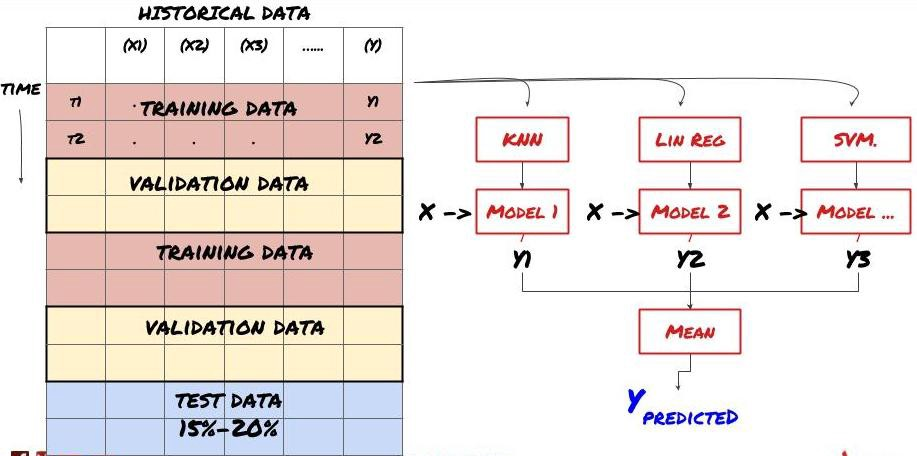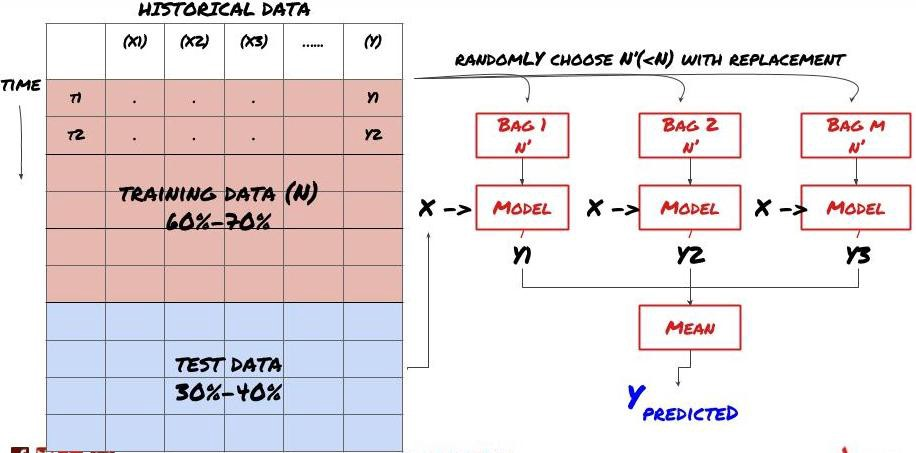Bagging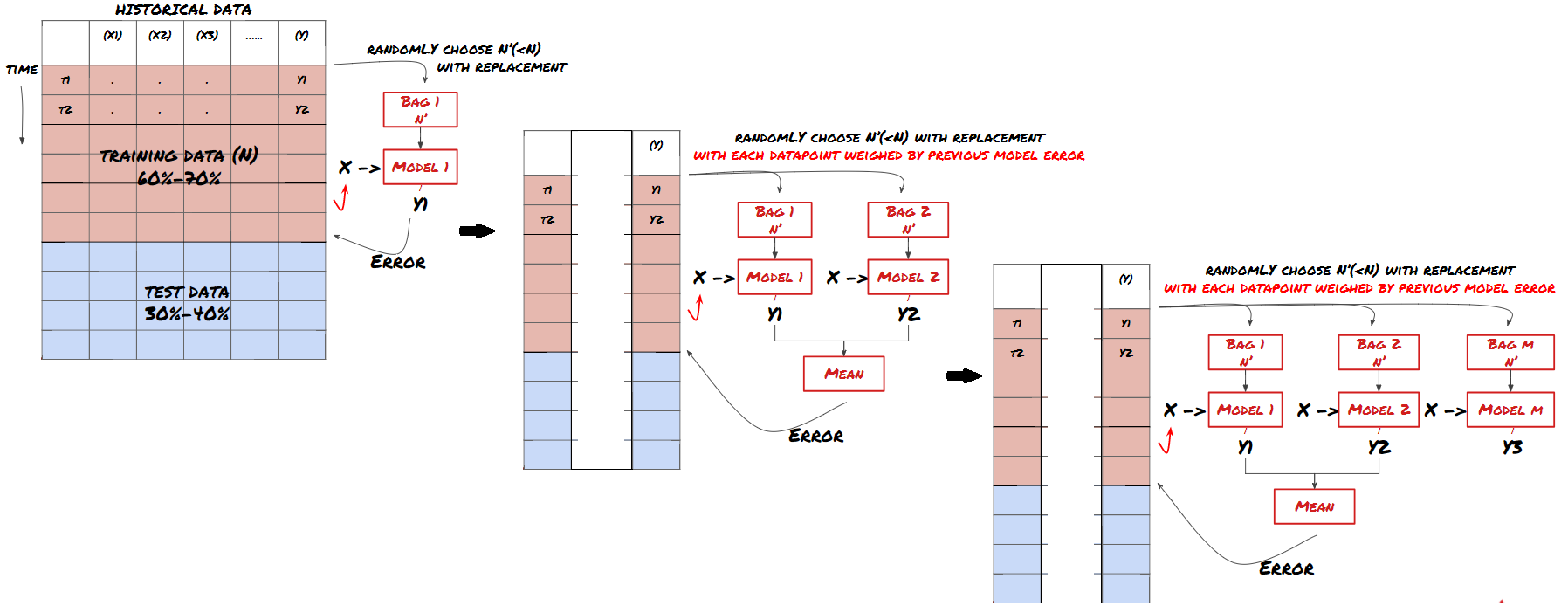Boosting

basis_y_pred_ensemble = (basis_y_trees + basis_y_svr +
basis_y_knn + basis_y_regr)/4Mean squared error: 0.02
Variance score: 0.95

到目前为止,我们已经积累了很多知识和信息.让我们快速回顾一下：

• 解决你的问题

• 收集可靠的数据和清理数据

• 将数据拆分为训练，验证和测试集

• 创建特征并分析其行为

• 根据行为选择合适的训练模型

• 使用训练数据训练你的模型并进行预测

• 检查验证集上的性能并重新优化

• 验证测试集的最终性能

• 开发基于预测模型的信号以识别交易方向

• 开发识别开平仓的具体策略

• 执行系统以识别仓位和价格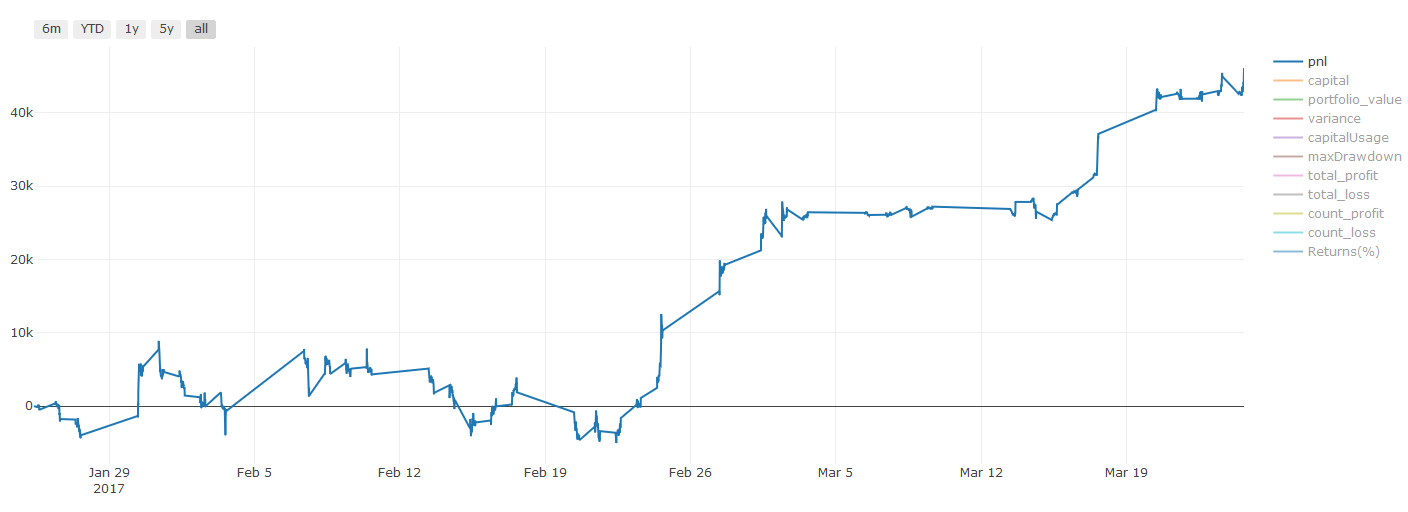该做什么和不该做什么

• 以所有力量避免过度拟合！

• 不要在每个数据点后重新训练：这是人们在机器学习开发中犯的常见错误。如果你的模型需要在每个数据点之后重新训练，那么它可能不是一个非常好的模型。也就是说，它需要定期重新训练，只需要以合理的频率进行训练（例如，如果进行日内预测，则在每周结束时重新训练）

• 避免偏差，尤其是前瞻性偏差：这是模型不起作用的另一个原因,确保你未使用未来的任何信息。大多数情况下，这意味着，不要使用目标变量Y作为模型中的特征。在回溯测试期间，你可以使用它，但在实际运行模型时将无法使用，这将使你的模型无法使用。

• 警惕数据挖掘偏差：由于我们正在尝试对我们的数据进行一系列建模以确定是否适合，如果没有其特殊的原因，请确保你运行严格的测试以将随机模式与可能发生的实际模式分开。例如，线性回归很好地解释了向上趋势模式，单很可能会成为较大随机游走的一小部分！

避免过度拟合

• 过度拟合是交易策略中最危险的陷阱

• 一个复杂的算法可能在回测中表现非常出色，但在新的看不见的数据上却惨遭失败,这个算法并没有真正揭示数据的任何趋势，也没有真正的预测能力。它非常适合它所见的数据

• 让你的系统尽可能简单。如果你发现自己需要大量复杂功能来解释数据，那么你可能会过度拟合

• 将你的可用数据划分为训练和测试数据，并始终在使用模型进行实时交易之前验证真实的样本数据的性能。

More

congcong009 很棒的文章，思路和总结上适合初学练手